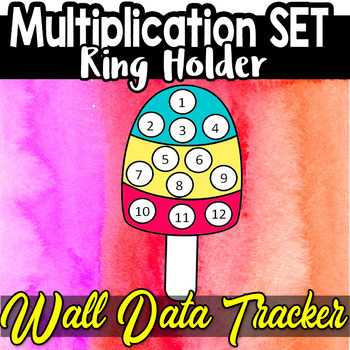DID YOU KNOW:
Seamlessly assign resources as digital activities

Learn how in 5 minutes with a tutorial resource. Try it Now

Learn More# Multiplication Facts Data Bulletin Rings Flash Cards Homework Take home2nd - 6th
Subjects
Standards
Resource Type
Formats Included
• PDF

### Description

Why do make rings instead of flash cards in my class?

•Rings can be reused if laminated

•They can be sent home and not lost

•Easy to store

•Easy to print on colorful paper and add new facts as students learn them

•Can be used when working on other math concepts

These are in a small set that can be printed on only one paper if need or

can be printed one set as a time (Example : 2 multiplication facts sheet only) .

0-12

Let me know if anything is missing Will update if needed. Thank you.

Total Pages
N/A
Teaching Duration
N/A
Report this Resource to TpT
Reported resources will be reviewed by our team. Report this resource to let us know if this resource violates TpT’s content guidelines.

### Standards

to see state-specific standards (only available in the US).
Understand division as an unknown-factor problem. For example, find 32 ÷ 8 by finding the number that makes 32 when multiplied by 8.
Determine the unknown whole number in a multiplication or division equation relating three whole numbers. For example, determine the unknown number that makes the equation true in each of the equations 8 × ? = 48, 5 = __ ÷ 3, 6 × 6 = ?.
Interpret products of whole numbers, e.g., interpret 5 × 7 as the total number of objects in 5 groups of 7 objects each. For example, describe a context in which a total number of objects can be expressed as 5 × 7.